# Dot Situation

Reasoning is one of the most important section in a Competitive exam. A good score in Reasoning test can lead you to score very good marks in competitive exams. This can be achieve only if you have a very good reasoning skills. Basically reasoning is divided into few sections according to logic and type. Dot Situation is one of them.

Dot Situation relate to a activity in which we have to find a common characteristic between a dot placed in between some figure and enclosed area by the same figures.

This section deals with the questions in which a group of geometrical shapes with a dot is given and it is asked to find from the given options, the one which is similar to the given group of geometrical shapes. Each of the four (or more) answer figures is composed of a cluster of the same type of geometrical shapes (that the given in the question figure) and the correct answer figure is the one which contains an area common to the shapes that have been marked by the dot in the question figure.

Let us consider an example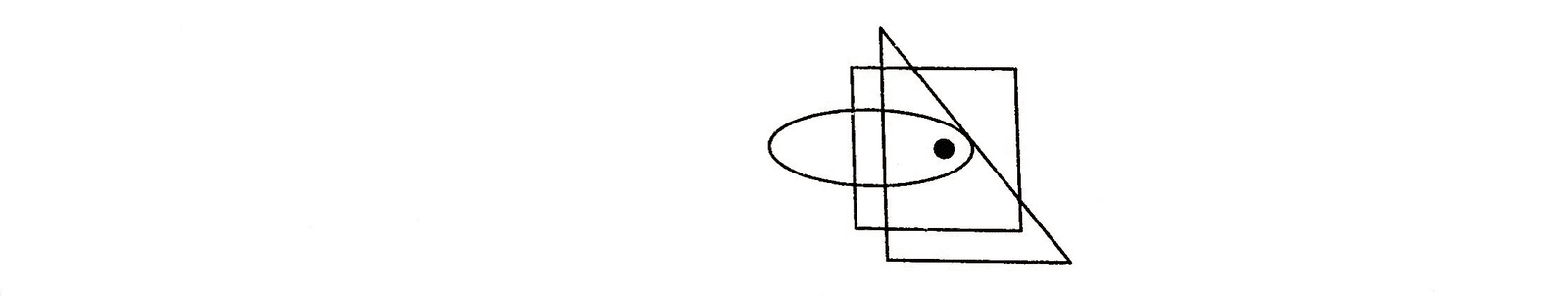In the above figure, a group of three geometrical shapes i.e., a square, a triangle and an oval is given. A dot is placed such that it is common to all the three, so another group of geometrical shapes similar to the one shown above is that which contains an area common to all the three shapes as shown below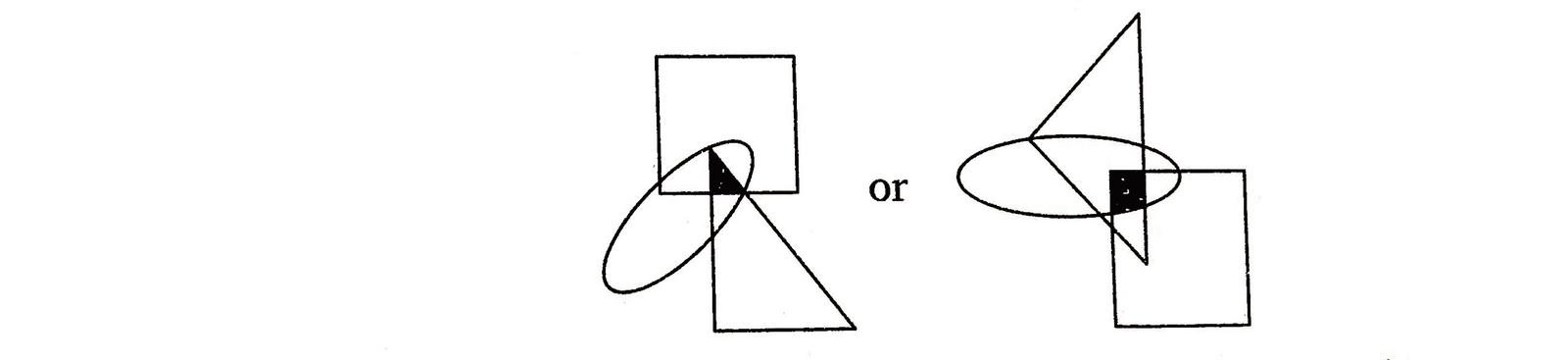Any other such group having a region common to all the three shapes holds a similar relationship with the group of geometrical shapes given above with the dot.

## Let us see some illustrative examples given below to have a better idea about the type of questions asked

Directions (Example Nos. 1-3)In the following questions, one or more dots are placed in the figure marked as (X). The figure is followed by four alternatives marked as (1), (2), (3) and (4). One out of these four options contains region(s) common to the circle, square, triangle, similar to that marked by the dot in figure (X).

E.g 1 Select the figure which satisfies the same conditions of placement of the dots as in Figure-X.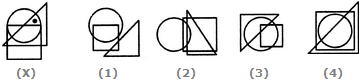Solution: (3) In figure (X), the dot is placed in the region which is common to the circle and triangle. Now, we have to search similar common region in the four options. Only in figure (3), we find such a region which is common to the circle and triangle.E.g 2 Select the figure which satisfies the same conditions of placement of the dots as in Figure-X.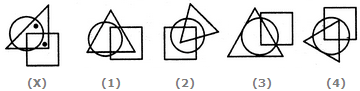Solution: (1) One dot occupies the region which is common to the circle and triangle and the other dot occupies the region which is common to the triangle and square. Out of all the answer figures, only answer figure (1) possesses a region which is common to the circle and triangle and a region which is common to the triangle and square.E.g 3 Select the figure which satisfies the same conditions of placement of the dots as in Figure-X.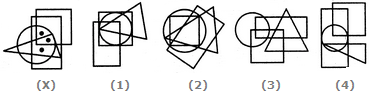Solution: (2) One of the three dots occupies the region which is common to the circle, rectangle and triangle; another dot occupies the region which is common to the triangle, circle, rectangle and square and the third dot occupies the region which is common to the circle, rectangle and square.

These three characteristics as shown by these three dots are found in figure (2). It possesses region which is common to the circle, rectangle and triangle, a region which is common to the triangle circle, rectangle, and square and a region which is common to the circle, rectangle and square.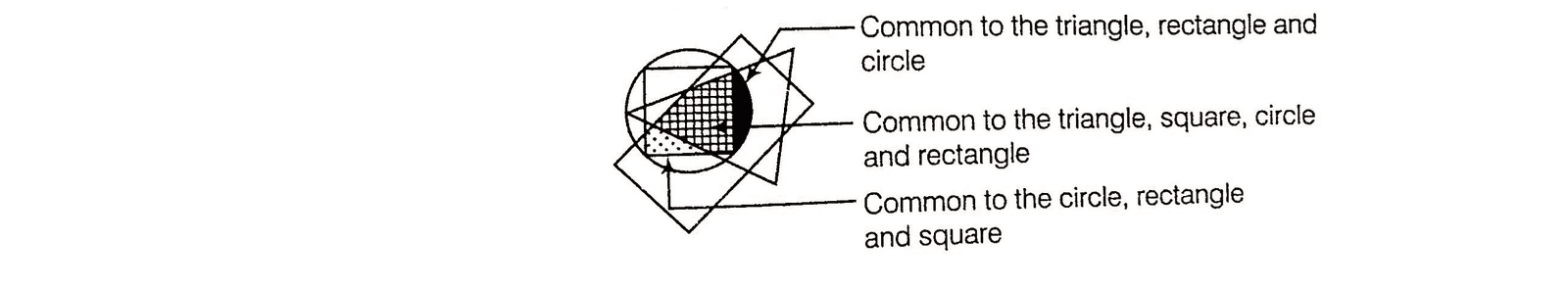## TIPS on cracking Non Verbal Reasoning Questions on Dot Situation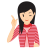Few Important things to Remember

Time is a very important thing in every exams. You should complete your exam within the time frame. But in a competitive exam, question are for testing your calculation ability within a given time frame. They tests, how quickly a student can solve a question paper. This is the reason so many students couldn’t finish their paper within given time. But if you need to solve your government or bank or any other question paper quickly then you should use tricks of reasoning and Dot Situation.

Tip# : In Dot Situation problems, simply find the figure that contains all the sections as the dots in the original figure

Question: Question: Select the figure which satisfies the same conditions of placement of the dots as in Figure-X.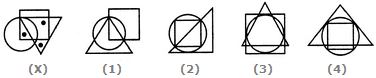Solution:

In fig. (X), one of the dots lies in the triangle alone, another dot lies in the region common to the square and the triangle only and the third dot lies in the region common to the circle and the square only. In each of the figures (1), (2) and (4), there is no region common to the square and the triangle only. Only fig. (3) consists of all the three types of regions.